### 5.1.1 Newtonian Limit

The Newtonian model obstructs wave solution, due to the instantaneous interaction. Following Refs. [28,29,30], we consider a model whose action propagates at infinite speed (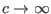). This is compatible with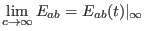, whereis an arbitrary function of time.

We define the Newtonian potential as(71)

On substituting into Eq. (65a), we get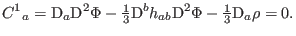(72)

In a spatial infinity, we obtain the Poisson equation of the Newtonian potential: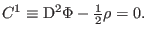(73)

Equation (65a) generalizes the gravitoelectric as the Newtonian force in the gradient of the relativistic energy density.

Moreover, Eq. (66a) gives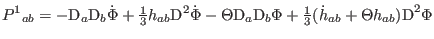(74)

In the Newtonian theory, we could not find the temporal evolution of the Newtonian potential.

Ashkbiz Danehkar
2018-03-26